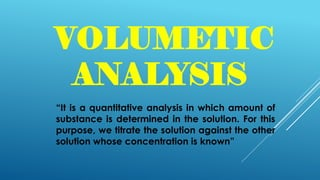Successfully reported this slideshow.

# Volumetric Analysis×

# Volumetric Analysis

It is a quantitative analysis in which amount of substance is determined in the solution. For this purpose, we titrate the solution against the other solution whose concentration is known
Practical work
Titration
Types
Titrant
titrand
Procedure
Calculation
Precaution

It is a quantitative analysis in which amount of substance is determined in the solution. For this purpose, we titrate the solution against the other solution whose concentration is known
Practical work
Titration
Types
Titrant
titrand
Procedure
Calculation
Precaution

### Volumetric Analysis

1. 1. Prof. Sohaib Hasnain Punjab Group of Colleges, Faisalabad CHEMISTRY
2. 2. VOLUMETIC ANALYSIS “It is a quantitative analysis in which amount of substance is determined in the solution. For this purpose, we titrate the solution against the other solution whose concentration is known”
3. 3. SOME IMPORTANT TERMS: STANDARD SOLUTION : The solution whose concentration is already known Concentration : It is relative amount of solute in a solution STRENGTH : when the amount of substance dissolved in a solution is expressed in gram per dm3
4. 4. WHAT IS TITRATION “IT IS THE PROCESS OF FINDING THE CONCENTRATION OF UNKNOWN SOLUTION” Types • Acid Base titration • Redox titration • Compelxometric titration
5. 5. APPARATUS • Burette • Pipette • Conical flask • Burette stand • Pipette sucker • Beaker • Measuring Flask
6. 6. DIFFERENCE B/W TITRANT AND TITRAND Titrant : The solution which is taken in burette Titrand : The solution which is taken in Conical Flask
7. 7. PROCEDURE • Fill the titrant in burette • take 10 cm3 titrand in conical flask • Add 2-3 drops of indicator to the flask • Now add the solution from burette to the flask drop wise • End point shows some change in color • Note the final reading • Repeat the experiment for three time
8. 8. CALCULATION Avarage = 10+10+10 3 = 10 cm3 No. of Obs Initial value Final value Volume used 1 0.0 10.0 10.0 cm3 2 10.0 20.0 10.0 cm3 3 20.0 30.0 10.0 cm3
9. 9. CHEMICAL EQUATION ACID + BASE → SALT + WATER HCl + NaOH → NaCl + H2O MOLAR RATIO For acid n1= 1 mol For base n2 = 1 mol
10. 10. MOLARITY FORMULA 𝑀1𝑉1 𝑛1 = 𝑀2𝑉2 𝑛2 Strength = Molarity ×Molar mass
11. 11. PRECAUTIONS • Use lab coat and gloves • Wash the apparatus before and after use • Always use funnel for adding solution in burette • Always use sucker for pipetting out the solution •